# The level

The level of temperature of the liquid in a thermometer is 24.32°C lower than the boiling point of water. What is the thermometer reading? Show your answer by drawing and shading the thermometer.

t =  75.68 °C

### Step-by-step explanation: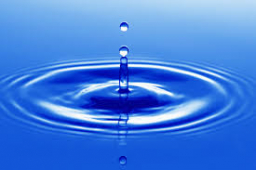Did you find an error or inaccuracy? Feel free to write us. Thank you!## Related math problems and questions:

• Lower temperature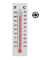The level of temperature of the liquid in a thermometer is 24.32°C lower than the boiling point of water. What is the thermometer reading? Show your answer by drawing and shading the thermometer.
• Change in temperatureStarting temperature is 21°C, the highest temperature is 32°C. What is the change in temperature?
• ThermometerThe thermometer showed -12 degrees Celsius in the morning then the temperature rises by 4 degrees and later again increased by 2 degrees at the evening has fallen by 5 degrees and then falls 3 degrees. What end temperature does the thermometer show?
• The temperature 11The temperature on Sunday was 4°C. The temperature dropped 8°C on Monday and twice as much on Tuesday. What is the temperature on Tuesday?
• The temp rangeWhat is the range in temperatures if the high temperatures is 67 degrees Celsius and the low temperature is -25 degrees Celsius?
• Ground levelThe temperature at ground level in Helsinki is 20°C lower than in London. The temperature in a basement flat in Helsinki is 5°C below the temperature at ground level. If it is -29°c in the basement, what is the temperature in London?
• Melting point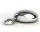The melting point of mercury is -36°F and its boiling point is 672°F. What is the difference between the boiling point and the melting point?
• The temperature 10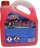The temperature in a freezer is -15°C, and it increases by 3°C.
• Temperature 13Work out the temperature after each change. The temperature starts at six °C and falls by 13°C. The temperature starts at two °C and falls by eight °C
• WeatherWeather. At 5:00 am, the temperature was 28°C, and at noontime, it rose by 9°C. By 4:00 pm, it dropped 3°C, and by nighttime, it was 4°C less. What is the room temperature this time?
• Thermometer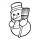The thermometer showed -3°C in the morning. Then the temperature was increased by 1°C again increased by 1°C and then decreased by 1°C and then decreased by 4°C. Which terminal temperature thermometer shows?
• Temperature difference 2The temperature in London on new year’s day is -2 degree Celsius. The temperature in Moscow on the same day is -14 degree Celsius, what is the temperature difference between the two cities?
• The temperature 16The temperature at 6 am was 21 degrees Fahrenheit by noon, and the temperature dropped 32 degrees Fahrenheit. What was the temperature at noon?
• Now isNow is 5°C morning. What will the temperature be if i. It rises by 12°C ii. It falls by 15°C
• Mixing Celsius and FahrenheitAdd up three temperatures: 5°F +6°F +0°C
• NoontimeIn the morning temperature was 110°F. By noontime, it has gone up by 15°F. What was the noon temperature? (Give your answer in °F)
• The temperature 14The temperature is 12°C lower than 4 hours ago. a. What is the average temperature change per hour? b. Write an integer equation for the situation.